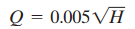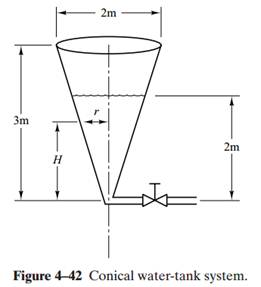# Consider the conical water-tank system shown in Figure 4–42. The flow through the valve is… 1 answer below »

Consider the conical water-tank system shown in Figure 4–42. The flow through the valve is turbulent and is related to the head H bywhere Q is the flow rate measured in m3/sec and H is in meters.

Suppose that the head is 2 m at t=0. What will be the head at t=60 sec?

Figure 4–42